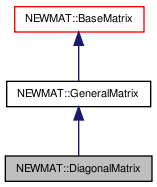# NEWMAT::DiagonalMatrix Class Reference

`#include <newmat.h>`

Inheritance diagram for NEWMAT::DiagonalMatrix:[legend]

## Detailed Description

Definition at line 795 of file newmat.h.

List of all members.

## Public Member Functions

DiagonalMatrix ()
~DiagonalMatrix ()
DiagonalMatrix (ArrayLengthSpecifier)
DiagonalMatrix (const BaseMatrix &)
DiagonalMatrix (const DiagonalMatrix &gm)
void operator= (const BaseMatrix &)
DiagonalMatrixoperator= (Real f)
DiagonalMatrixoperator= (const DiagonalMatrix &m)
Real & operator() (int, int)
Real & operator() (int)
Real operator() (int, int) const
Real operator() (int) const
Real & element (int, int)
Real & element (int)
Real element (int, int) const
Real element (int) const
MatrixType Type () const
LogAndSign LogDeterminant () const
Real Trace () const
void GetRow (MatrixRowCol &)
void GetCol (MatrixRowCol &)
void GetCol (MatrixColX &)
void NextRow (MatrixRowCol &)
void NextCol (MatrixRowCol &)
void NextCol (MatrixColX &)
GeneralMatrixMakeSolver ()
void Solver (MatrixColX &, const MatrixColX &)
GeneralMatrixTranspose (TransposedMatrix *, MatrixType)
void ReSize (int)
void ReSize (const GeneralMatrix &A)
Real * nric () const
MatrixBandWidth BandWidth () const
void operator+= (const DiagonalMatrix &M)
void operator-= (const DiagonalMatrix &M)
void operator+= (Real f)
void operator-= (Real f)
void swap (DiagonalMatrix &gm)

## Private Member Functions

GeneralMatrixImage () const

## Constructor & Destructor Documentation

 NEWMAT::DiagonalMatrix::DiagonalMatrix ( )

Definition at line 799 of file newmat.h.

Referenced by Image().

 NEWMAT::DiagonalMatrix::~DiagonalMatrix ( )

Definition at line 800 of file newmat.h.

 NEWMAT::DiagonalMatrix::DiagonalMatrix ( ArrayLengthSpecifier m )

Definition at line 67 of file newmat4.cpp.

 NEWMAT::DiagonalMatrix::DiagonalMatrix ( const BaseMatrix & M )

Definition at line 145 of file newmat4.cpp.

 NEWMAT::DiagonalMatrix::DiagonalMatrix ( const DiagonalMatrix & gm )

Definition at line 803 of file newmat.h.

## Member Function Documentation

 MatrixBandWidth NEWMAT::DiagonalMatrix::BandWidth ( ) const` [virtual]`

Reimplemented from NEWMAT::BaseMatrix.

Definition at line 445 of file newmat4.cpp.

 Real NEWMAT::DiagonalMatrix::element ( int m ) const

Definition at line 748 of file newmat6.cpp.

 Real NEWMAT::DiagonalMatrix::element ( int m, int n ) const

Definition at line 733 of file newmat6.cpp.

 Real & NEWMAT::DiagonalMatrix::element ( int m )

Definition at line 741 of file newmat6.cpp.

 Real & NEWMAT::DiagonalMatrix::element ( int m, int n )

Definition at line 725 of file newmat6.cpp.

Referenced by NEWMAT::SortSV(), NEWMAT::SVD(), and NEWMAT::tred2().

 void NEWMAT::DiagonalMatrix::GetCol ( MatrixColX & mrc ) ` [virtual]`

Implements NEWMAT::GeneralMatrix.

Definition at line 246 of file newmat3.cpp.

 void NEWMAT::DiagonalMatrix::GetCol ( MatrixRowCol & mrc ) ` [virtual]`

Implements NEWMAT::GeneralMatrix.

Definition at line 236 of file newmat3.cpp.

 void NEWMAT::DiagonalMatrix::GetRow ( MatrixRowCol & mrc ) ` [virtual]`

Implements NEWMAT::GeneralMatrix.

Definition at line 229 of file newmat3.cpp.

 GeneralMatrix * NEWMAT::DiagonalMatrix::Image ( ) const` [private, virtual]`

Reimplemented from NEWMAT::GeneralMatrix.

Definition at line 788 of file newmat4.cpp.

 LogAndSign NEWMAT::DiagonalMatrix::LogDeterminant ( ) const` [virtual]`

Reimplemented from NEWMAT::GeneralMatrix.

Definition at line 651 of file newmat8.cpp.

 GeneralMatrix* NEWMAT::DiagonalMatrix::MakeSolver ( ) ` [virtual]`

Reimplemented from NEWMAT::GeneralMatrix.

Definition at line 829 of file newmat.h.

 void NEWMAT::DiagonalMatrix::NextCol ( MatrixColX & mrc ) ` [virtual]`

Reimplemented from NEWMAT::GeneralMatrix.

Definition at line 260 of file newmat3.cpp.

 void NEWMAT::DiagonalMatrix::NextCol ( MatrixRowCol & mrc ) ` [virtual]`

Reimplemented from NEWMAT::GeneralMatrix.

Definition at line 257 of file newmat3.cpp.

 void NEWMAT::DiagonalMatrix::NextRow ( MatrixRowCol & mrc ) ` [virtual]`

Reimplemented from NEWMAT::GeneralMatrix.

Definition at line 254 of file newmat3.cpp.

 Real* NEWMAT::DiagonalMatrix::nric ( ) const

Definition at line 834 of file newmat.h.

 Real NEWMAT::DiagonalMatrix::operator() ( int m ) const

Definition at line 183 of file newmat6.cpp.

 Real NEWMAT::DiagonalMatrix::operator() ( int m, int n ) const

Definition at line 175 of file newmat6.cpp.

 Real & NEWMAT::DiagonalMatrix::operator() ( int m )

Definition at line 71 of file newmat6.cpp.

 Real & NEWMAT::DiagonalMatrix::operator() ( int m, int n )

Definition at line 63 of file newmat6.cpp.

 void NEWMAT::DiagonalMatrix::operator+= ( Real f )

Reimplemented from NEWMAT::GeneralMatrix.

Definition at line 840 of file newmat.h.

 void NEWMAT::DiagonalMatrix::operator+= ( const DiagonalMatrix & M )

Definition at line 838 of file newmat.h.

Referenced by operator+=().

 void NEWMAT::DiagonalMatrix::operator-= ( Real f )

Reimplemented from NEWMAT::GeneralMatrix.

Definition at line 841 of file newmat.h.

 void NEWMAT::DiagonalMatrix::operator-= ( const DiagonalMatrix & M )

Definition at line 839 of file newmat.h.

Referenced by operator-=().

 DiagonalMatrix& NEWMAT::DiagonalMatrix::operator= ( const DiagonalMatrix & m )

Definition at line 806 of file newmat.h.

 DiagonalMatrix& NEWMAT::DiagonalMatrix::operator= ( Real f )

Reimplemented from NEWMAT::GeneralMatrix.

Definition at line 805 of file newmat.h.

 void NEWMAT::DiagonalMatrix::operator= ( const BaseMatrix & X )

Definition at line 408 of file newmat6.cpp.

Referenced by operator=().

 void NEWMAT::DiagonalMatrix::ReSize ( const GeneralMatrix & A ) ` [virtual]`

Reimplemented from NEWMAT::GeneralMatrix.

Definition at line 332 of file newmat4.cpp.

 void NEWMAT::DiagonalMatrix::ReSize ( int nr )
 void NEWMAT::DiagonalMatrix::Solver ( MatrixColX & mrc, const MatrixColX & mrc1 ) ` [virtual]`

Reimplemented from NEWMAT::GeneralMatrix.

Definition at line 497 of file newmat2.cpp.

 void NEWMAT::DiagonalMatrix::swap ( DiagonalMatrix & gm )

Definition at line 842 of file newmat.h.

Referenced by NEWMAT::swap().

 Real NEWMAT::DiagonalMatrix::Trace ( ) const` [virtual]`

Reimplemented from NEWMAT::BaseMatrix.

Definition at line 550 of file newmat8.cpp.

 GeneralMatrix * NEWMAT::DiagonalMatrix::Transpose ( TransposedMatrix * , MatrixType mt ) ` [virtual]`

Reimplemented from NEWMAT::GeneralMatrix.

Definition at line 57 of file newmat5.cpp.

 MatrixType NEWMAT::DiagonalMatrix::Type ( ) const` [virtual]`

Implements NEWMAT::GeneralMatrix.

Definition at line 431 of file newmat4.cpp.

The documentation for this class was generated from the following files:

 newmat11b Generated Mon May 9 04:54:19 2016 by Doxygen 1.6.3